# OOF2: The Manual

## 8.5. Adding New Outputs

Warning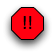This section has not yet been updated for OOF2 version 2.1. A partial discussion of the differences between the 2.0 and 2.1 extension APIs may be found at http://www.ctcms.nist.gov/oof/oof2/property_api_21.html.

`Outputs` in OOF2 are operations performed on `Mesh` data. The results can be sent to the graphics window and displayed in a contour plot, or analyzed on the Analysis Page.

The outputs that the user sees come in three flavors:

• Scalar outputs are just numbers, to be plotted or analyzed.

• Aggregate outputs are quantities like `Fields` and `Fluxes` that can't be plotted directly (because they generally have too many components) but can still be analyzed.

• Position outputs are two dimensional vectors that determine where scalar outputs are displayed in contour plots.

Figure 8.1 shows how scalar and position outputs appear in the GUI.

Figure 8.1. Output Widgets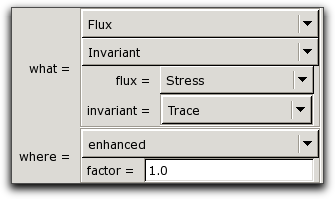Two output widgets, from the Layer Editor window. The top widget, labelled `what`, lists scalar outputs and determines what will be plotted in the graphics window. The lower widget, labelled `where`, lists position outputs, which are two dimensional vectors.

Under the hood, the scalar, aggregate, and position outputs are built by combining `Output` objects. Each `Output` object performs a simple operation on a set of input values and creates a set of output values. Simple `Outputs` are chained together, with the output of one connected to the input of another, to create more complicated `Outputs`. (One `Output` object can be used in many different Output chains.) Only those `Outputs` that are registered with the GUI are directly available to the user.

`Outputs` have parameters which govern their behavior. `Output` parameters use the same `Parameter` classes that are used in `PropertyRegistrations`.

OOF2 includes predefined `Outputs` that can be used by themselves or combined with new `Outputs`. The predefined `Outputs` can evaluate the components and invariants of `Fields` and `Fluxes` and compute energies and strains. For the details, consult the source code in `SRC/engine/IO/outputClones.py` and `SRC/engine/IO/outputDefs.py`.

Creating a new `Output` involves the following steps:

• Write a callback function. This is what does the actual computation. See Output for the details.

• Invoke the `Output` constructor, specifying the type of the `Output`, the types of its inputs (if any), its parameters (if any), and its callback function.

• Connect the `Output` to its inputs, if any, by calling `Output.connect`.

• Set parameters in the inputs, if desired. `Parameters` which have fixed values in the `Output` definition will not be settable by the user. To make a `Parameter` settable by the user, leave its value alone (or set it to `None`). Use `Output.findParam` or `Output.resolveAlias` to gain access to an `Output`'s parameters, or those of its connected inputs.

• If any of the inputs have parameters that are not specified in advance, these need to given aliases so that they can be used in scripts and the GUI. See `Output.aliasParam` for the details.

• If the `Output ` is to appear in the GUI, it must be registered by calling `definePositionOutput`, `defineScalarOutput`, or `defineAggregateOutput`.

### 8.5.1. Examples

Here are a few example `Output` definitions, extracted from the OOF2 source code. The examples illustrate all of the important features of the `Output` class.

#### 8.5.1.1. Evaluating a Field

The first example returns `Field` values as `OutputVal` instances. It's copied from `SRC/engine/IO/outputClones.py`.

```def _field(mesh, elements, coords, field):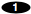ans = []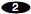for element, ecoords in zip(elements, coords):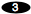ans += element.outputFields(mesh, field, ecoords)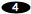return ans

FieldOutput = output.Output(
name = "field",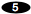callback = _field,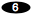otype = outputval.OutputValPtr,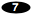params = [meshparameters.FieldParameter("field", outofplane=1)],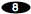) ```
 This is the callback function. All callbacks have `mesh`, `elements`, and `coords` arguments. `elements` is a list of the mesh `Elements` within which the output is to be computed. `coords` is a list of lists of `MasterCoords` specifying the computation points within each `Element`. Any additional callback arguments are either inputs or parameters, with names determined by the input and parameter lists in the `Output` constructor. Here `field` is a `Parameter` value. The result of the computation is a flat list of `OutputVal` objects, one for each coordinate in `coords`. The `zip` call here matches up each `Element` to the list of coordinates at which the output should be evaluated within the `Element`. `element` is an `Element`, and `ecoords` is a list of `MasterCoords`. `Element:outputFields` returns a list of `OutputVals` containing `Field` values at the specified points within the given `Element`. The list is appended to results already obtained. This specifies the name of the `Output`. It will be used to when setting parameters and connecting this `Output` to others. It will not be used in scripts or the user interface. This is just the name of the callback function defined above. This specifies the type of the quantities computed by this `Output`. This states that the `Output` has one parameter, named `field`, whose value is a `Field`.

To make the `FieldOutput` available in scripts and the GUI, `SRC/engine/IO/outputDefs.py` contains the following lines:

```from oof2.common.IO import output
from oof2.engine.IO import outputClones
output.defineAggregateOutput('Field:Value', outputClones.FieldOutput) ```

`defineAggregateOutput` states that this `Output` computes an aggregate object (such as all of the components of a `Field` or `Flux`), as opposed to a scalar object. `'Field:Value'` categorizes the output — the GUI will refer to it as the `Value` item in the `Field` menu.

#### 8.5.1.2. Connecting Inputs

Note that the `FieldOutput` has no inputs! It reads its data from the mesh, not from any other `Output`. The next example, `ComponentOutput`, has both a parameter and an input, but doesn't read data from the mesh.

```def _component(mesh, elements, coords, field, component):if field:comp = field.getIndex(component)return [f[comp] for f in field]return []

import types

ComponentOutput = output.Output(
name = "component",
callback = _component,
otype = types.FloatType,inputs = [outputval.OutputValParameter('field')],params = [meshparameters.FieldIndexParameter('component')]) ```
 The callback here has the usual required `mesh`, `elements`, and `coords` arguments, although it doesn't use them. `field` is an input list of `OutputVal` objects, and `component` is a parameter value. It's possible that the list of values could be empty. The next line assumes that it's not empty, so we have to check. The `component` is passed in in a user-friendly string form (such as `'x'` or `'yz'`). This line asks the `OutputVal` class to convert the string into a computer-friendly `FieldIndex` object. (`field` is used just because it's an `OutputVal` of the correct type. Its actual value isn't important here.) Different kinds of `OutputVal` interpret the strings differently. This computes and returns a list of components of the input `OutputVal` objects. Components of an `OutputVal` are floating point numbers, so the output type of `ComponentOutput` is `FloatType`. Inputs are specified by a list of `Parameters`, although they don't actually have values the same way that `Parameters` do. The input `Parameter` specifies the name and type of the input. In this case, the input is called `'field'` and is a list of `OutputVals` (or `OutputValPtrs`). The `Output` has a single parameter, named `'component'`, whose value is a string representation of a component index.

Note that despite the name of its input, `'field'`, the `ComponentOutput` can extract components from any kind of `OutputVal`, not only ones containing `Fields`. The built-in OOF2 outputs use `ComponentOutput` on `Fields`, `Fluxes`, and other multicomponent quantities.

The `FieldOutput` and `ComponentOutput` can be combined into a `FieldCompOutput`, which extracts a given component of a given `Field`, by using `Output.connect`. First, a copy of the `ComponentOutput` is made, giving it a new name at the same time:

`FieldCompOutput = ComponentOutput.clone(name="field component") `

`FieldOutput` is connected to the input named `'field'` of the `FieldCompOutput`:

`FieldCompOutput.connect("field", FieldOutput) `

`connect` automatically makes a copy of its argument, so we don't have to worry about cloning `FieldOutput` here.

#### 8.5.1.3. Managing Output Parameters

At this stage, `FieldCompOutput` has two parameters and no inputs. (The one input of the original `ComponentOutput` has been filled by the `FieldOutput` that was connected to it.) The original `FieldOutput` parameter, named `'field'`, and the `ComponentOutput` parameter, named `'component'`, still remain. `'component'` is a direct parameter of `FieldCompOutput`. It can be accessed by the `findParam` method, e.g:

`FieldCompOutput.findParam('component').value = 'x' `

Setting a parameter's value like this gives it a permanent value, as far as `Outputs` are concerned. The user will not be able to change the value, and the field-component `Output` has been changed into an field-x-component `Output`.

The `'field'` parameter of the original `FieldOutput` can be accessed in a similar, but not identical way. The `FieldCompOutput` has no parameter called `'field'`, so we can't use `FieldCompOutput.findParam('field')`, and the original `FieldOutput` has been cloned, so its `'field'` parameter isn't the same one that `FieldCompOutput` uses. The parameter can be accessed by specifying both the name of the input and the parameter in the call to `findParam`:

`FieldCompOutput.findParam('field:field').value = Displacement `

Here the first `field` is the name of the input, and the second is the name of the parameter of that input.

Assuming that we want the user to be able to choose the `Field` and the component, we don't actually want to use `findParam` and set the parameter values. However, we don't want the user to see an ugly name like `'field:field'`. For one thing, parameter names are used as Python variable names in scripts, and Python variable names can't contain colons. The function `Output.aliasParam` assigns a new name to an `Output` parameter, like this:

`FieldCompOutput.aliasParam('field:field', 'field') `

Now `FieldCompOutput` has a parameter called `'field'` instead of `'field:field'`. Note that there's no conflict between the aliased parameter named `'field'` and the input named `'field'`. The confusion is always resolved by the context.

`Output.findParam` never considers aliases, so the call `FieldCompOutput.findParam('field')` will fail (it will raise a `KeyError` exception). To retrieve an aliased parameter, use `Output.resolveAlias` instead. If `resolveAlias` can't find an alias, it looks for a parameter, so the following three function calls are equivalent:

```param = FieldCompOutput.resolveAlias('field')
param = FieldCompOutput.resolveAlias('field:field')
param = FieldCompOutput.findParam('field:field') ```

Aliases are only known to the `Outputs` that create them. In the example above, the `FieldOutput` containing the original `'field'` parameter doesn't know that it's been aliased. However, if `FieldCompOutput` were to be used as an input to another `Output`, like this:

`SomeOtherOutput.connect('otherinput', FieldCompOutput) `

then the `'field'` parameter could be accessed by

`param = SomeOtherOutput.resolveAlias('otherinput:field') `

using the alias still stored in `FieldCompOutput`.

#### 8.5.1.4. Handling Multiple Parameters and Inputs

The examples above all use at most one parameter and one input. `Outputs` can have as many parameters and inputs as they like. The `PointSumOutput` from `SRC/engine/IO/outputClones.py` is used to add the Displacement to the original position of a node. It's actually a little more general than that — it takes two input streams which are lists of `Coord` or `Point` objects, multiplies them by scalar factors, and adds them together.

```from oof2.SWIG.common import coord
from oof2.common import primitives
from oof2.common.IO import output
from oof2.common.IO import parameter

def _pointSum(mesh, elements, coords, point1, point2, a, b):ans = [a*f+b*s for f,s in zip(point1, point2)]return ans

PointSumOutput = output.Output(
name="point sum",
callback=_pointSum,
otype=(coord.Coord, primitives.Point),inputs=[coord.CoordParameter("point1"), coord.CoordParameter("point2")],params=[parameter.FloatParameter("a", default=1.0),parameter.FloatParameter("b", default=1.0)]
) ```
 The callback arguments include the usual `mesh`, `elements`, and `coords`, although they're not used here. `point1` and `point2` are the names of the inputs, and `a` and `b` are the names of the parameters. This line does all the work. It can be written in such a simple way because inputs are flat lists of data. Because the input (see) accepts either `Coords` or `Points`, the output can be either `Coords` or `Points`. There are two inputs, named `point1` and `point2`. The `CoordParameter` class accepts either a `Coord` or a `Point`. The two parameters, named `'a'` and `'b'` have been given default values of `1.0`, which is the value that will be displayed in the GUI if the variable hasn't been set yet.

The `PointSumOutput` is used in `SRC/engine/IO/outputDefs.py` to add the displacement to the node position. The displacement values come from `displacementOutput` and the position values come from `posOutput`, which won't be explicitly discussed here. The connection looks like this:

```enhancedPosition = outputClones.PointSumOutput.clone(
name='enhanced position',
params=dict(b=1),tip='Exaggerated displacement field.')enhancedPosition.connect('point1', displacementOutput)enhancedPosition.connect('point2', outputClones.posOutput)enhancedPosition.aliasParam('a', 'factor', default=1.0,tip='Displacement multiplier.') ```
 Parameters can be set when cloning an `Output`. The `params` argument is a dictionary. The dictionary keys are the names (or aliases) of the parameters to be set. Parameters set in this way remain set. The user will not have a chance to modify them. Most of the examples have omitted the optional `tip` argument. When creating or cloning `Outputs`, it's possible to specify a `tip` string, which will appear as a help string in the GUI, and a `discussion` string, which will appear in the manual. These lines connect the preexisting `displacementOutput` and `posOutput` `Outputs` to the inputs of the `PointSumOutput` clone. This line shows that `aliasParam` can be used to change the name of a local parameter, as well as parameters in the inputs. Here the non-descriptive parameter `'a'` is renamed `'factor'` and given a default value and a `tip` string.

At this point, the `enhancedPosition` `Output` has one parameter, `'factor'`, and no inputs. It produces a list of node positions, with displacement exaggerated by the given amount. The `actualPosition` `Output` is now simply created by cloning `enhancedPosition` and setting the enhancement factor to 1.0:

```actualPosition = enhancedPosition.clone(
name='actual position',
params=dict(factor=1.0),
tip='Displaced position.') ```

Finally, both `enhancedPosition` and `actualPosition` are made available in the GUI by calling `definePositionOutput`:

```output.definePositionOutput('actual', actualPosition)
output.definePositionOutput('enhanced', enhancedPosition) ```

### 8.5.2. `PropertyOutputs`

Output quantities that need to use information from the material `Properties` of the `Mesh` are treated differently because they need to hook into existing `Property` instances and because many different `Properties` may contribute to any given `Output`. Outputs such as this are defined by creating an instance of a `PropertyOutputRegistration` subclass, and listing the name of the subclass in a `Property`'s `PropertyRegistration` `outputs` list. The `PropertyOutputRegistration` will automatically create `Output` objects. When these `Outputs` are evaluated, the `Property`'s `output` function will be called, with a `PropertyOutput` object as one of its arguments. `Property::output` can use the `PropertyOutput` object to find out which output is being computed, and to access the output's parameters.

Clear? Here's an example, illustrating the `Energy` output and how it's computed by the `Elasticity` property. Some of the code has been slightly simplified for brevity, but the source files have been indicated for anyone interested in all the details.

First, an `Enum` class is created to distinguish the different types of energy (in `SRC/engine/IO/outputDefs.py`):

```from oof2.common import enum

class EnergyType(enum.EnumClass("Total", "Elastic", ...)):
pass ```

The same file then defines a `ScalarPropertyOutputRegistration`, because energy is a scalar quantity:

```from oof2.SWIG.engine.IO import propertyoutput

propertyoutput.ScalarPropertyOutputRegistration(
"Energy",
parameters=[enum.EnumParameter("etype", EnergyType, default="Total")]
) ```

The string `"Energy"` is the name by which the output will be known both in the user interface and in the `Property` code. The parameter name `"etype"` will also appear in the user interface and the code. It's important that the parameter does not have an assigned value! The Output mechanism assumes that parameters with values are not going to be set by the user. Instead, the parameter has its `default` set, which provides a value to be displayed in the GUI, without actually fixing the `Parameter`'s value.

Note that the output registration does not contain any information about what `Energy` is, or how it's computed. That's the `Properties`' job. `Elasticity`'s `output` function looks like this (in `SRC/engine/property/elasticity/elasticity.C`):

```void Elasticity::output(const FEMesh *mesh,
const Element *element,
const PropertyOutput *output,
const MasterPosition &pos,
OutputVal *data)
const
{
const std::string &outputname = output->name();
if(outputname == "Energy") {
std::string etype = output->getEnumParam("etype");
if(etype == "Total" || etype == "Elastic") {
ScalarOutputVal *edata = dynamic_cast<ScalarOutputVal*>(data);
SymmMatrix strain(3);
const Cijkl modulus = cijkl(mesh, element, pos);
findGeometricStrain(mesh, element, pos, strain);
SymmMatrix stress(modulus*strain);
double e = 0;
for(int i=0; i<3; i++) {
e += stress(i,i)*strain(i,i);
int j = (i+1)%3;
e += 2*stress(i,j)*strain(i,j);
}
*edata += 0.5*e;
}
}
} ```

See the `Property::output` discussion for another example, with annotations.

Finally, the `Elasticity` `PropertyRegistration` needs to indicate that `"Energy"` is one of the outputs that it can compute. There are many types of elasticity, and each has its own registration. Here's the one for isotropic elasticity, from `SRC/engine/property/elasticity/iso/iso.spy`:

```from oof2.engine import propertyregistration

propertyregistration.PropertyRegistration(
name = "Mechanical:Elasticity:Isotropic",
subclass = IsoElasticityProp,
...
outputs=["Energy"],
...) ```

`IsoElasticityProp` is a subclass of `Elasticity`, so because `PropertyRegistration` indicates that `IsoElasticityProp` can compute `"Energy"`, when a `Material` contains `IsoElasticityProp`, `Elasticity::output` will be called to compute it.

Property outputs can never use other `Outputs` as inputs, but they can, in principle, be used as inputs for other `Outputs`. However, there's no machinery to make this easy to do, yet.

### 8.5.3. Orientation Map Formats

TODO: Write this section.

 Ok, we lied. The example is copied from an old version of the source code. The given example is very inefficient because the `+=` inside the loop is continuously reallocating and copying the `ans` list. See the actual code in `SRC/engine/IO/outputClones.py` for a more efficient but less obvious scheme.

 Note that the type is `OutputValPtr`, not `OutputVal`. That's because `OutputVal` is a swigged C++ class. swig creates two Python classes for each C++ class. One, with a name ending in `Ptr` (e.g, `OutputValPtr`) is a pointer (of sorts) to the C++ object, and is used to refer to an object that was created in C++. The other Python class has no `Ptr` suffix (e.g, `OutputVal`) and is used when an object was created directly in Python. In the `FieldOutput` callback, `Element::outputFields` returns `OutputVals` created by the C++ code.

In general, in `otype` specifications, it's safe to use the `Ptr` form of a swigged class if you're not sure which is correct. The type checking mechanism allows subclasses of the given type. `Ptr` will always work because the non-`Ptr` version of a class is derived from the `Ptr` version.

 It's also possible to change the `Output`'s `tip` and `discussion` strings, which have been omitted here.

 The `Coord` and `Point` classes are equivalent, except that `Coord` is a swigged C++ class, and `Point` is a pure Python class. There are circumstances in which the overhead of calling C++ from Python makes using a Python class more efficient.

 The parameter dictionary can also be written as `params={'b' : 1}`. This form would be required if a full parameter name containing colons were being used, e.g `params={'input:b' : 1}`, because the `dict` form requires that the keywords be legal Python variable names.

 The default value setting is redundant in this case, because the default value of `'a'` was already set to 1 when `PointSumOutput` was created.